##### Pre-Calculus For Dummies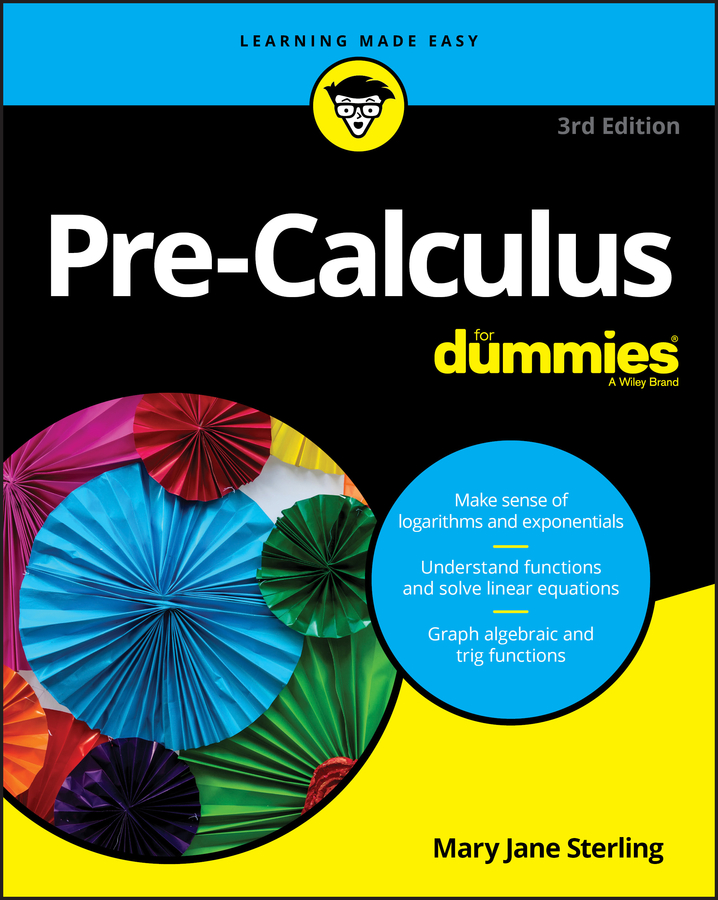When your pre-calculus teacher asks you to find the partial sum of a geometric sequence, the sum will have an upper limit and a lower limit. The common ratio of partial sums of this type has no specific restrictions.

You can find the partial sum of a geometric sequence, which has the general explicit expression of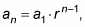by using the following formula: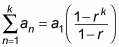For example, to find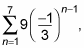1. Find a1 by plugging in 1 for n.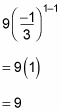2. Find a2 by plugging in 2 for n.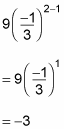3. Divide a2 by a1 to find r.

For this example, r = –3/9 = –1/3. Notice that this value is the same as the fraction in the parentheses.

You may have noticed that 9(–1/3)n – 1 follows the general formula for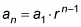(the general formula for a geometric sequence) exactly, where a1 = 9 and r = –1/3. However, if you didn't notice it, the method used in Steps 1–3 works to a tee.

4. Plug a1, r, and k into the sum formula.

The problem now boils down to the following simplifications: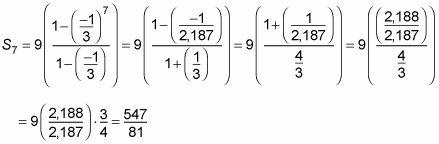Geometric summation problems take quite a bit of work with fractions, so make sure to find a common denominator, invert, and multiply when necessary. Or you can use a calculator and then reconvert to a fraction. Just be careful to use correct parentheses when entering the numbers.